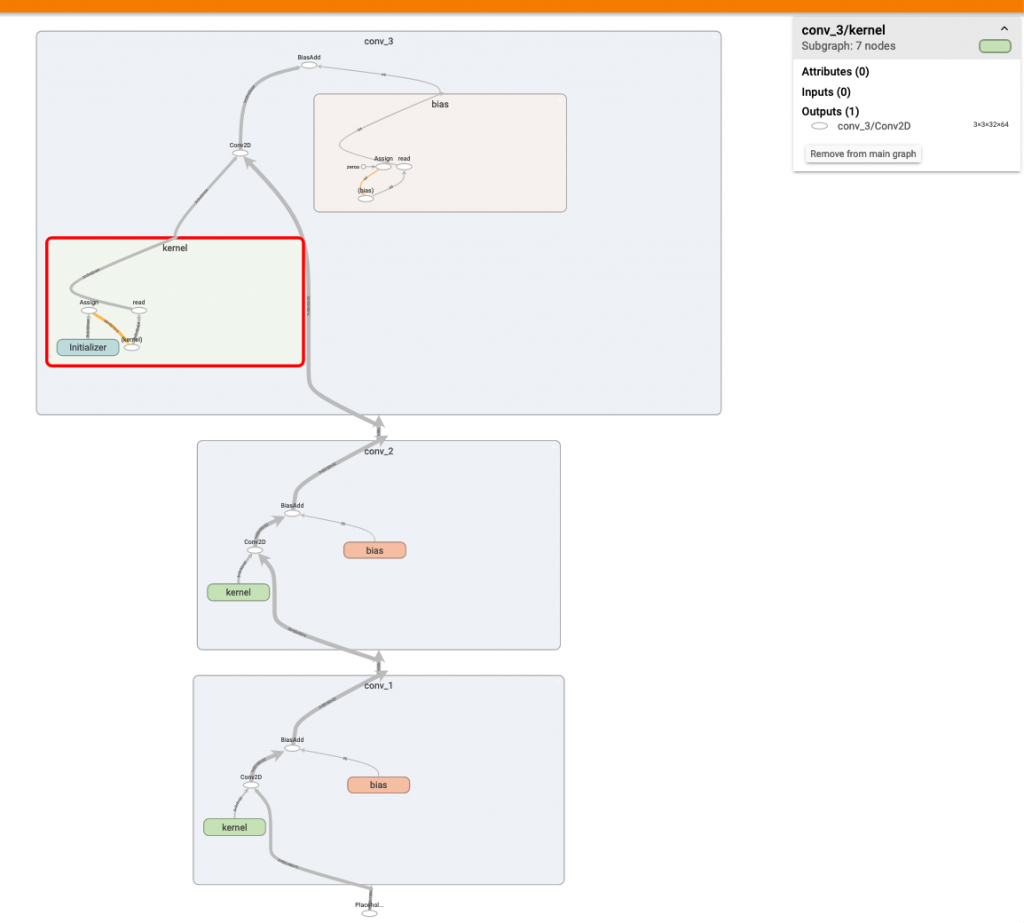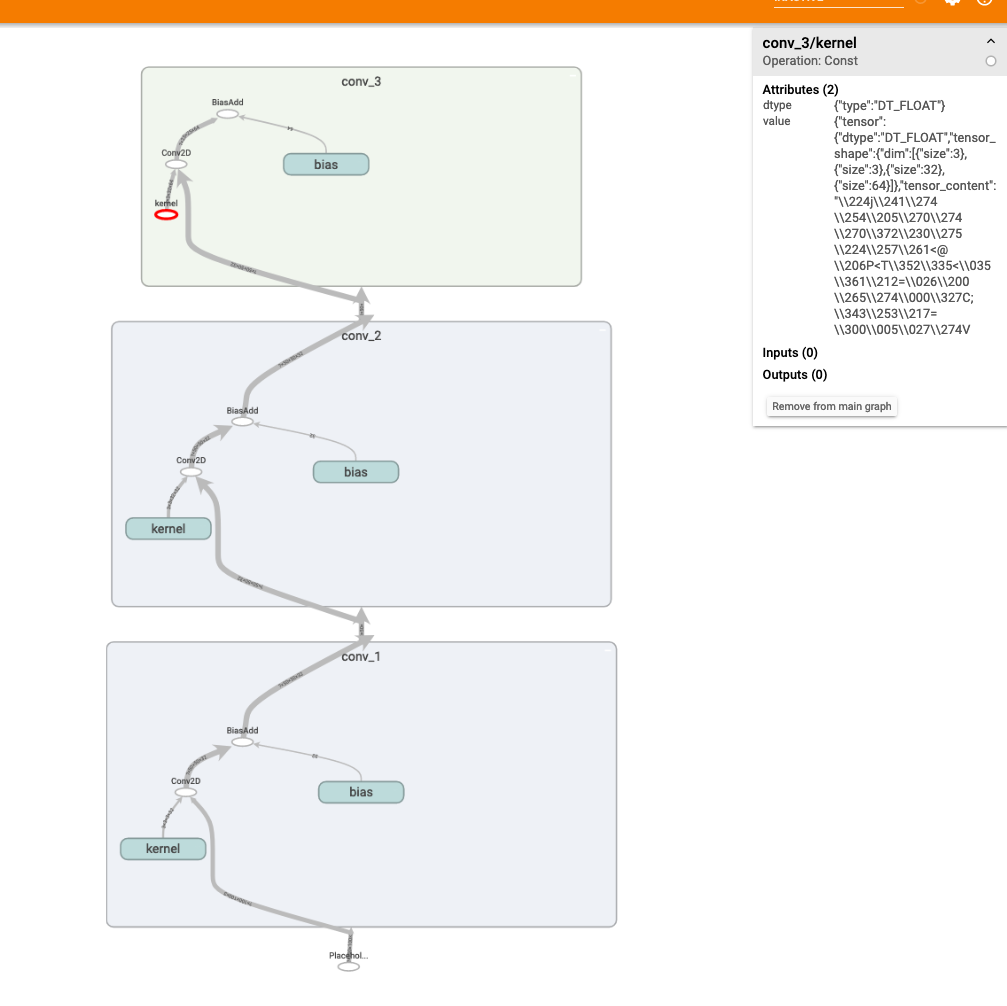DAY 8
0
Google Developers Machine Learning

How to Train Your Model 訓模高手：我的 Tensorflow 個人使用經驗系列文系列 第 8 篇

graph_def = tf.get_default_graph().as_graph_def()
with gfile.FastGFile(MODEL_PB, 'rb') as f:
tf.import_graph_def(graph_def, name='')

pbtxt 也是很類似，我們要靠 google.protobuf.text_format 來讀取。

graph_def = tf.get_default_graph().as_graph_def()
with gfile.FastGFile(MODEL_PBTXT, 'rb') as f:
tf.import_graph_def(graph_def, name='')

google.protobuf.text_format.ParseError: 1563:10 : Message type "tensorflow.GraphDef" should not have multiple "versions" fields.

versions {   producer: 38 }

graph_def = tf.GraphDef()
with gfile.FastGFile(MODEL_PBTXT, 'rb') as f:
tf.import_graph_def(graph_def, name='')

Bonus: 動腦時間：
Q: 最後一個範例中，為什麼我import FROZEN_PBTXT 用default graph就沒有問題？
.
.
.
.
.
.
.
.
.
.
.
A: 因為之前 tf.graph_util.convert_variables_to_constants() 在產生時，指定的 output node 不需要 vesrion 這個欄位，產生出來的 frozen graph 就把這個欄位丟掉了，因此就不會撞名啦！github原始碼